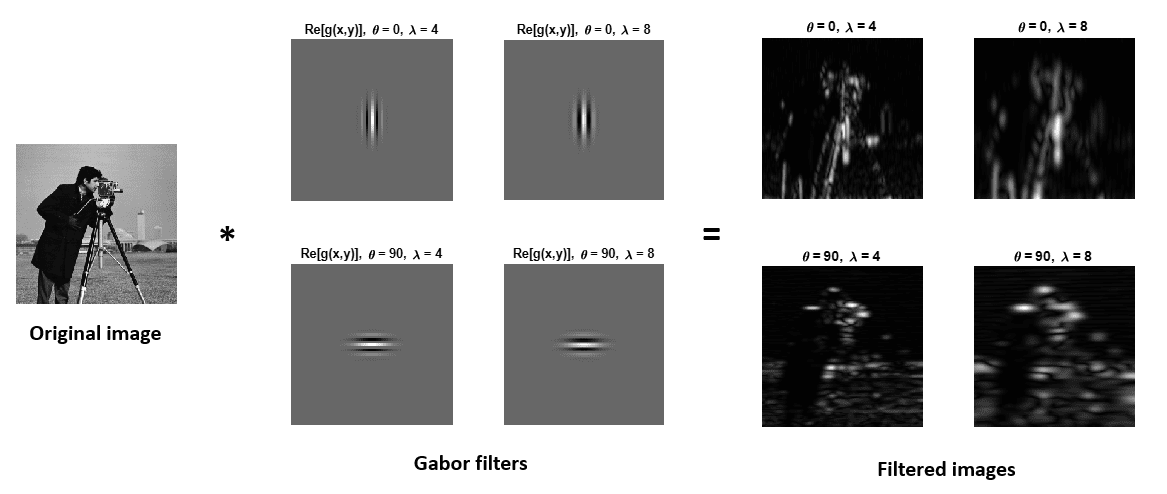## 1. Overview

In this tutorial, we’ll talk about the Gabor filters, a classic tool in image processing.

First, we’ll explain how to compute them. Then we’ll illustrate how to generate features for machine learning using Gabor filters.

## 2. What Are Gabor Filters?

A Gabor filter is a linear filter used in image processing for edge detection, texture classification, feature extraction and disparity estimation. It is a bandpass filter, i.e. it passes frequencies in a certain band and attenuates the other frequencies outside such band. A Gabor filter is a Gaussian modulated by a plane wave.

The convolution kernel can be expressed as:

(1)whereand. In Eq. 1is the wavelength of the plane wave,represents the orientation of the parallel stripes of the filter,is the standard deviation of the Gaussian component,is the aspect ratio that defines the ellipticity of the function support,is the phase of the plane wave.

The response matrixis obtained by convolving the original imagewith the Gabor filter:

(2)Several filters can be defined by changing the parameters,,,and. A Gabor filter bank is a set of Gabor filters with different parameters.

Different low-level features can be extracted from the original image via the convolution operation by varying the Gabor parameters. The following figure shows several filtered images obtained using the real parts of Gabor filters with different orientationsand wavelength:We can see that horizontal and vertical features are extracted withand, respectively. Furthermore, we can extract finer details with lower wavelength values.

The image analysis with Gabor filters well represents the perception in the human visual system.

## 3. Generating Features With Gabor Filters

There are several ways to extract features with Gabor filters. The most relevant ones are discussed below:

• The simplest way to generate features with Gabor Filters is to use the real or the imaginary part of the response matrix as a feature vector
• The phase of the response matrix can be taken as a feature vector since it contains relevant information about the edges
• The amplitude of the response matrix can be taken as a feature vector since it includes the frequency spectrum
• The squared sum of different wavelength responses with the same orientation can be taken as a feature vector. It represents the local energy in a particular direction

## 4. Conclusion

In this article, we reviewed the Gabor convolution filters, commonly used in image processing. Finally, we illustrated how to extract features from images using Gabor filters.